# How to Copy a Formula Down in Excel

Imagine filling in formulas into a thousand cells manually – that would be a nightmare. Thankfully, Excel allows you to copy formulas into other cells easily. This basic task can save you plenty of time. However, the way it’s done isn’t always clear to new Excel users.In this guide, we’ll explain how to copy a formula in Excel using three methods. We’ll also explain how to switch between relative and absolute formula references and answer common questions related to the topic.

## How to Copy a Formula Down in Excel by Dragging

You can quickly apply an Excel formula to adjacent cells by dragging the corner of the cell containing the formula. Follow the instructions below:

1. Click on the cell containing the formula you wish to copy.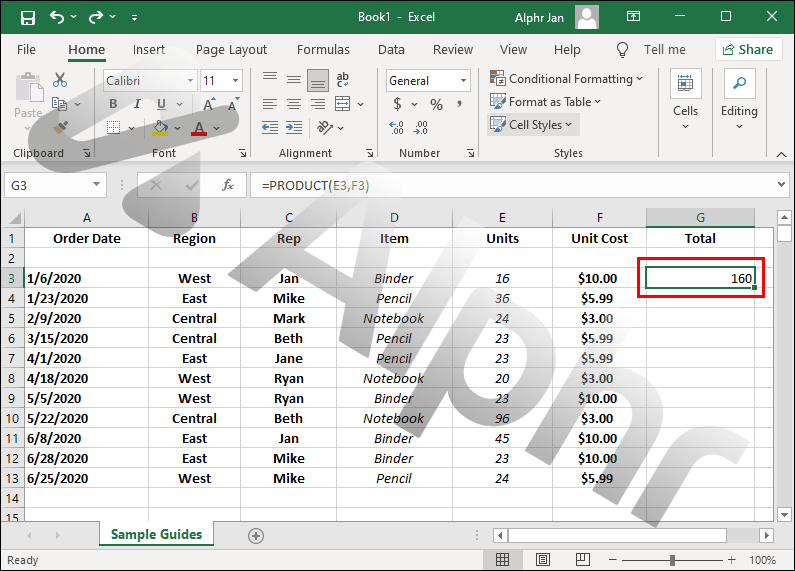2. Hover your cursor over the bottom right corner of the cell. Your cursor should turn into a plus sign.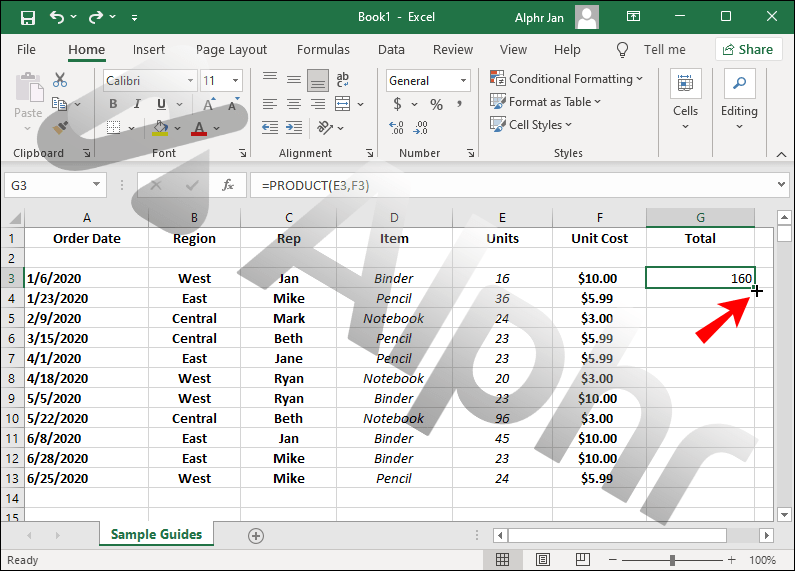3. Select and hold the bottom right corner of the cell, then drag it into the desired direction to select cells to fill.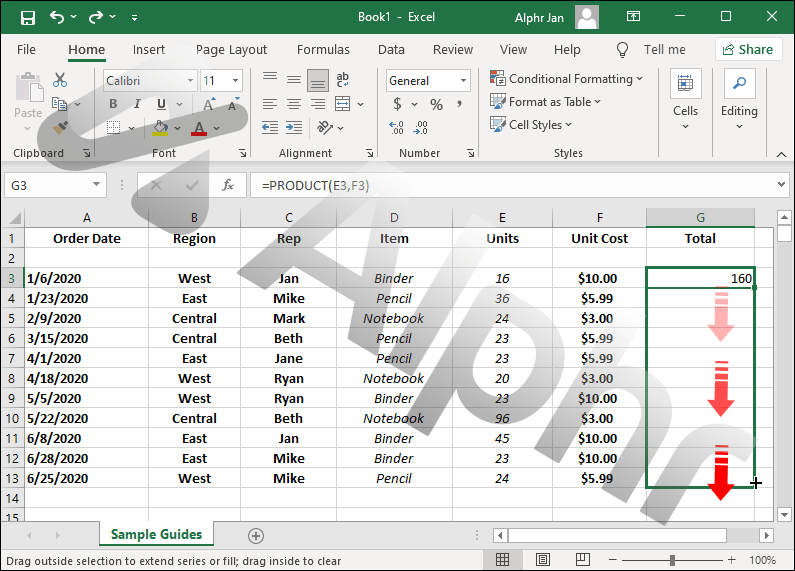4. Release the mouse. The formula will be applied to the selected cells automatically.If your cursor doesn’t turn into a plus sign and you can’t drag the cell, this feature might be hidden. Follow the steps below to enable it:

1. Click “File” at the top of your screen.2. Select “Options” from the dropdown menu.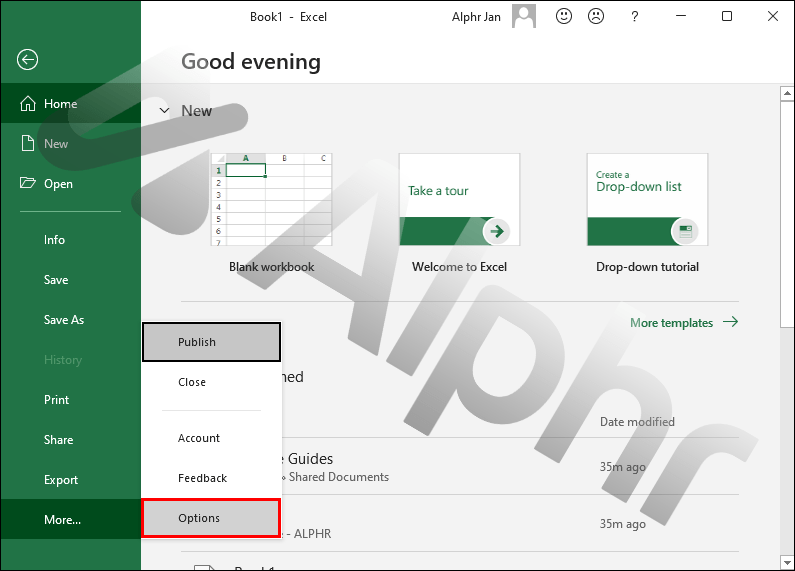4. Click “Enable fill handle and cell drag-and-drop option” under “Editing Options.”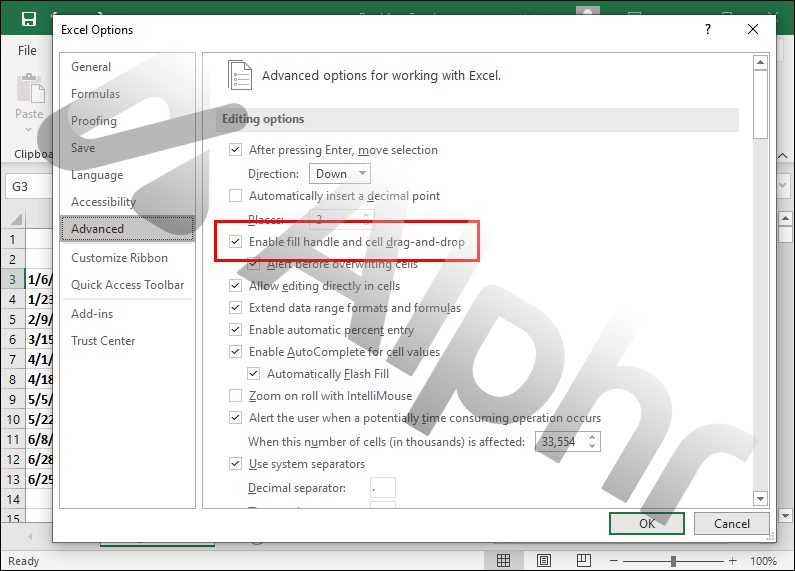## How to Copy a Formula Down in Excel Without Dragging

Sometimes, you may need to copy an Excel formula into non-adjacent cells, and the dragging method won’t work. In this case, you can select cells manually. Follow the steps below:

1. Click the cell containing the formula.2. Press the “Ctrl” and “C” keys simultaneously to copy the formula.3. Press and hold the “Ctrl” key, then click on the cells to which you wish to apply the formula.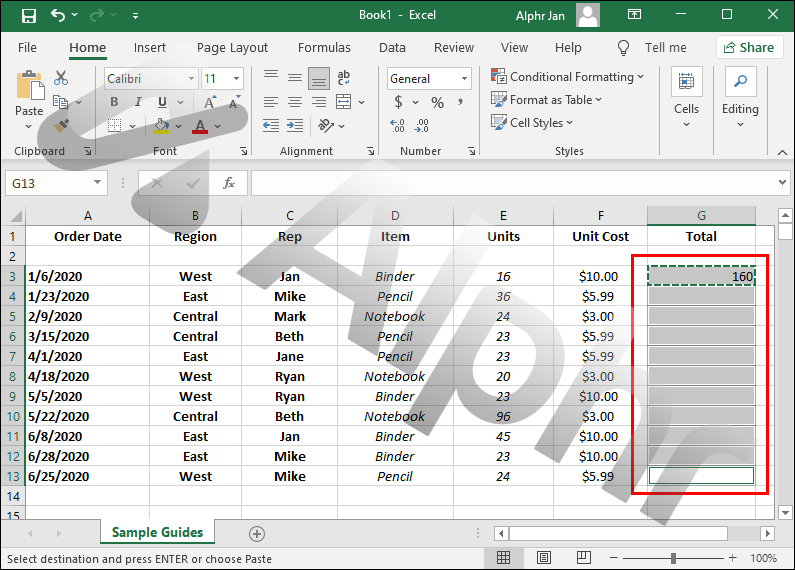4. Click an arrow next to “Paste” at the top of the document and select “Formula.” It will be applied to the selected cells automatically.If you need to quickly copy a formula down into an entire column, you can use the “Ctrl + D” shortcut. Follow the instructions below:

1. Select the cell with the formula.2. Click the index of the desired column (the letter at the top).3. Press the “Ctrl” and “D” keys simultaneously to apply the formula to the column.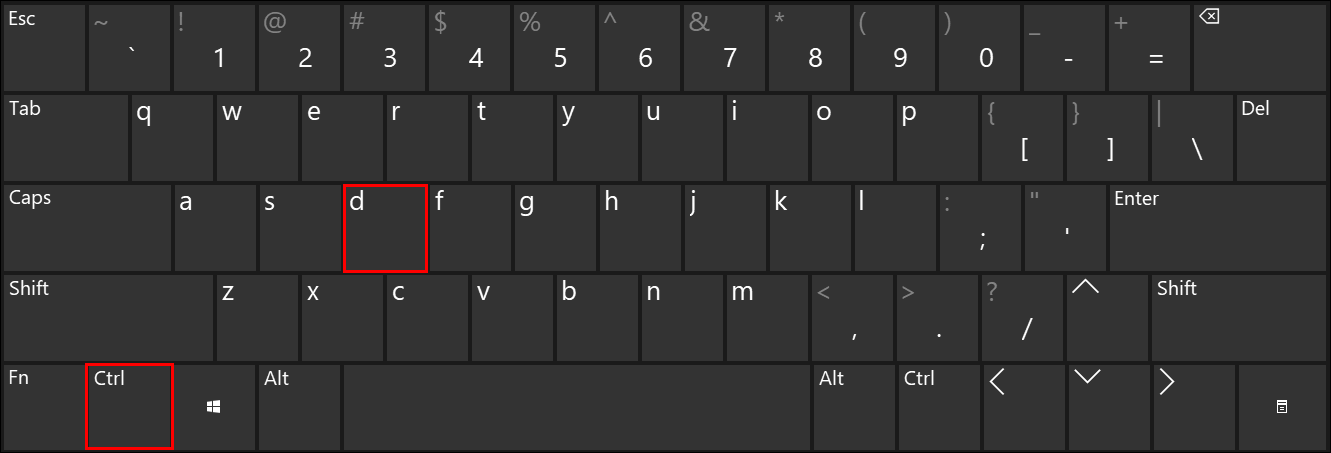Shortcuts also allow you to copy a formula into an entire row. Here’s how to do that:

1. Select a cell with the formula.2. Choose a row number on the left and highlight the whole row.
3. Press the “Ctrl” and “R” keys simultaneously.

## How to Copy a Formula Down in Excel Without Changing the Formula

By default, Excel formulas contain relative cell references. The formula values change depending on their relative position.

Let’s take a simple formula `=SUM(B1,C1)` as an example. If you copy it down, the next cells will contain formulas `=SUM(B2,C2)`, `=SUM(B3,C3)`, and `=SUM(B4,C4)`.

However, sometimes, you may need to fix some values in the formula.

Let’s assume you want to sum the B1 cell with each value in the C column. In this case, you should add a dollar sign `(\$)` in front of the column and row index. The formula should look like this: `=SUM(\$B\$1,C1)`, and the next cells will contain `=SUM(\$B\$1,C2)`, `=SUM(\$B\$1,C3)`, and `=SUM(\$B\$1,C4)` formulas. You can fix as many references as you want using this method.

### Can I Fix Only the Column Reference or Only the Row Reference in a Formula?

The dollar sign (\$) lets you fix chosen values in a formula. For example, if you wish the value in cell B3 to remain unchanged when copying the formula to other cells, add dollar signs in front of both the column and the row reference (\$B\$3).

However, sometimes you may need to fix only one reference. In this case, you should only add one dollar sign in front of either the column or the row reference. Like this:

• \$B3 – the row reference can change when the formula is copied into other cells, but the column reference can’t.

• B\$3 – the column reference can change, but the row reference is fixed.

### How Do I Copy the Cell Value Rather Than Its Formula?

By default, Excel copies the cell formula when using the “Ctrl + C” and “Ctrl + V” keyboard shortcuts. If you need to copy the cell value instead, follow the steps below:

1. Select the cell containing the value you wish to reproduce.

2. Use the “Ctrl + C” keyboard shortcut to copy the cell data.

3. Select the cells to paste the cell value into.

4. Click the arrow next to the “Paste” button at the top of your screen.

5. Select “Values.”

Now that you know how to copy formulas in Excel, your workflow should become much more efficient. Bear in mind that minor details in the instructions may differ depending on your Excel version. Keep the software up to date to ensure you don’t miss out on new features and always find the right guides.

Do you know any helpful tips for using Excel? Please share them in the comments section below.

Disclaimer: Some pages on this site may include an affiliate link. This does not effect our editorial in any way.

Todays Highlights